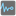• # Extra Task for Tester: Problem

## Question related to missionThe Most Frequent

Dear fellow mission solvers.

I just had a very good run today and learned quite a lot - until I came to this mission, which seems to have a hidden extra step at the tester, which returns my function as null! I don't get it why, because when I test it in VS code and here at the checkio code ditor everything works out, and at the checkout the two exercises get accepted.

Here is my attempt:

```function mostFrequent(a) {

let mf = 1;
let m = 0;
let item;
for (let i = 0; i < a.length; i++) {

for (let j=i; j < a.length; j++) {

if (a[i] == a[j]) {

m++; }

if (mf <  m) {

mf = m;
item = a[i];
}
}

m=0;

}
return item;
}
```

Maybe some of you know something about that third test which is invisible?

Cheers

Fabian